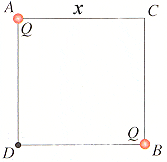# Electric Fields.

## Homework Statement

Two equal charges (Q = +0.80 nC) are situated at the diagonal corners A and B of a square of side x = 1.2 m as shown in the diagram. What is the magnitude of the electric field at point D?E=kQ/r^2

## The Attempt at a Solution

First I converted 0.80 nC into C and got 8.0e-10 C.

Using pythagorean's theorum I found 1.7 m as the distance between the two charges.

I then used Coulomb's law as follows:

9e9*8e-10/1.7^2 which gave me 2.5.

Since we want point D, I took the sin(45)2.5 + cos(45)2.5 and came up with 3.52 which was wrong.

Any ideas where I goofed?

The total field at D can just be considered as the field from particle A + the field from particle B. So the total equation is just E = 2 * k * Q / x^2

The total field at D can just be considered as the field from particle A + the field from particle B. So the total equation is just E = 2 * k * Q / x^2

Thanks for the reply. I took 2(9e9*8e-10)/1.7^2 and came up with 4.98 which did not seem to work.

square root of (2.5^2 + 2.5^2)?

square root of (2.5^2 + 2.5^2)?

Tried that earlier and it did not seem to work.

square root of (5^2 + 5^2) = 7.07?

square root of (5^2 + 5^2) = 7.07?

That answer was accepted. Thank you. If you have a minute, would you mind telling me how you came up with that?

1. electric field at D due to A is acting downward: magnitude 5
2. electric field at D due to B is acting to the left:magnitude 5
3. these two electric fields are vectors and are perpendicular to each other
4. if you are only interested in finding the magnitude of the resultant field,you can just apply pythagorean theorem.

Thank you, Leong.

make sure you use 1.2 m instead of 1.7 m because we find the field separately and individually; one by one and then only we find the overall/ resultant field.

glad to help and see that you really appreciate that...